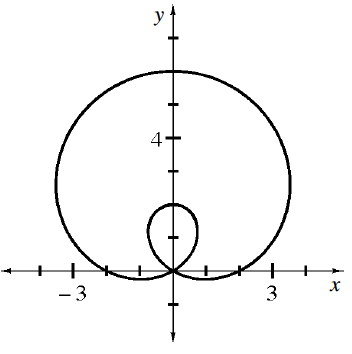### Home > CALC3RD > Chapter Ch9 > Lesson 9.4.3 > Problem9-137

9-137.

The graph below is called a limaçon. Use your conclusions from problem 9-128 to write its equation. Homework Help ✎$r = a + b\sin(θ)$

Notice that the points $(2, 0)$ and $(6, π/2)$ are on the graph.
Use these points and substitution to solve for the values of $a$ and $b$.